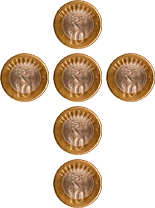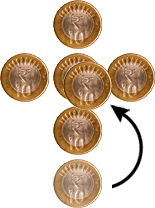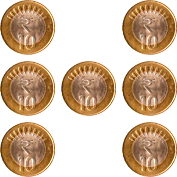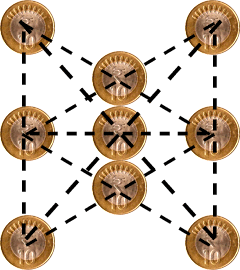1. Place six matchsticks in such a way that they all touch each other.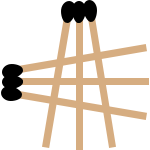2. Move only two matches and make four squares, one of them larger than the other three.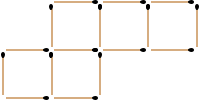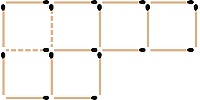3. Move four matches to form three equilateral triangles. [Hint. Who said three identical equilateral triangles?]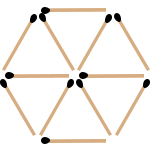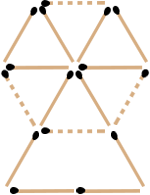4. Remove only four matches to leave exactly five identical squares.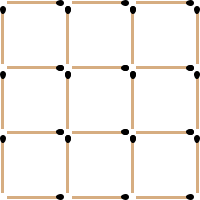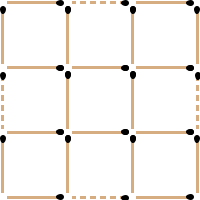5. In the above puzzle, what is the smallest number of matches you can remove to leave just two squares?

8 Match Sticks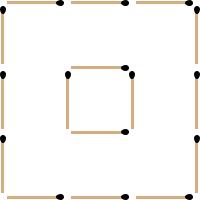6. Place six matchsticks in such a way that they form 4 triangles of the same size and shape. [Hint. Think 3 -D]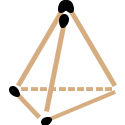7. Move only one matchstick each to make the following equations true.

1.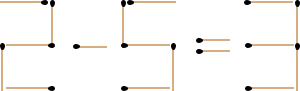2.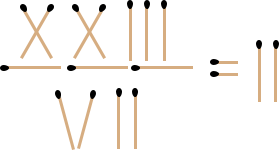3.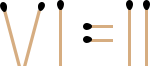1.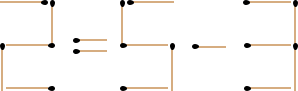2.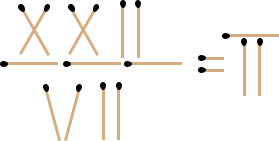3.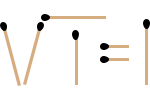8. Moving only 3 coins, how can you make this triangle point downwards?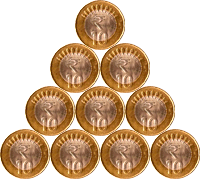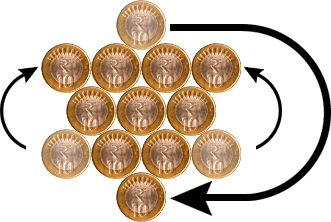9. Move just one coin to form two lines with four coins in each line.